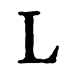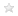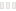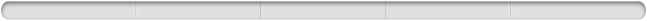Aquest lloc utilitza galetes per a oferir els nostres serveis, millorar el desenvolupament, per a anàlisis i (si no has iniciat la sessió) per a publicitat. Utilitzant LibraryThing acceptes que has llegit i entès els nostres Termes de servei i política de privacitat. L'ús que facis del lloc i dels seus serveis està subjecte a aquestes polítiques i termes.
Hide this

### Resultats de Google Books

Clica una miniatura per anar a Google Books.

S'està carregant…

# Reverse Mathematics: Proofs from the Inside Out (edició 2018)

## deJohn Stillwell (Autor)

 Membres Ressenyes Popularitat Valoració mitjana Converses 51 1 397,864(4) No n'hi ha cap This book presents reverse mathematics to a general mathematical audience for the first time. Reverse mathematics is a new field that answers some old questions. In the two thousand years that mathematicians have been deriving theorems from axioms, it has often been asked: which axioms are needed to prove a given theorem? Only in the last two hundred years have some of these questions been answered, and only in the last forty years has a systematic approach been developed. In Reverse Mathematics, John Stillwell gives a representative view of this field, emphasizing basic analysis--finding the "right axioms" to prove fundamental theorems--and giving a novel approach to logic. Stillwell introduces reverse mathematics historically, describing the two developments that made reverse mathematics possible, both involving the idea of arithmetization. The first was the nineteenth-century project of arithmetizing analysis, which aimed to define all concepts of analysis in terms of natural numbers and sets of natural numbers. The second was the twentieth-century arithmetization of logic and computation. Thus arithmetic in some sense underlies analysis, logic, and computation. Reverse mathematics exploits this insight by viewing analysis as arithmetic extended by axioms about the existence of infinite sets. Remarkably, only a small number of axioms are needed for reverse mathematics, and, for each basic theorem of analysis, Stillwell finds the "right axiom" to prove it. By using a minimum of mathematical logic in a well-motivated way, Reverse Mathematics will engage advanced undergraduates and all mathematicians interested in the foundations of mathematics.… (més)
 Membre: sanxiyn Títol: Reverse Mathematics: Proofs from the Inside Out Autors: John Stillwell (Autor) Informació: Princeton University Press (2018), 200 pages Col·leccions: La teva biblioteca Valoració:Etiquetes: No n'hi ha cap

## Detalls de l'obra

Reverse Mathematics: Proofs from the Inside Out de John Stillwell

 Afegit fa poc per anti-scholar, ugcCyTGLrPCgMkWbiyV, erathostenes, bunnyladame, dockevorkian, jpblib, ajtindall, Steve_WalkerNo n'hi ha cap
S'està carregant…Apunta't a LibraryThing per saber si aquest llibre et pot agradar.

No hi ha cap discussió a Converses sobre aquesta obra.

With Reverse Mathematics John Stillwell demonstrates the ideas and properties of Reverse Mathematics. I know it is a bit of a tautology to speak of it in this manner, so I will try to explain. Stillwell shows that mathematics had always been establishing axioms and then finding the results of those axioms. Reverse Mathematics goes in the other direction by taking a theorem and finding the axioms needed to prove it.

It is an interesting approach to Analysis, but I don’t really know all that much about Mathematical Analysis. I never took it in school, since I only got up to Calculus II in college. Therefore, there are several things that I find annoying, but that is only because I wasn’t paying attention the first time through. For instance, there is a portion where ZF is mentioned and I didn’t know what that was. The book might use a lot of acronyms but it usually explains what they mean before the author dives into the gist. This is important for when Stillwell decides to drop stuff like ACA and WKL and other such ideas.

In writing this book, it is clear from the text that the author wanted to establish a solid foundation for analysis given some advances in Logic. This is mentioned in the text itself. It doesn’t have any problems to solve or questions to answer.

The book is quite interesting as I mentioned before. It is rather short, but it is densely packed with ideas. It doesn’t have a glossary, choosing instead to jump straight to the Bibliography and Index. In that sense, I could say that the author dropped the ball, but it is possible that the editor thought it unnecessary for the target audience. Then again, you could always search for what he means by using the Internet. ()Has d'iniciar sessió per poder modificar les dades del coneixement compartit.
Si et cal més ajuda, mira la pàgina d'ajuda del coneixement compartit.
Títol normalitzatTítol originalTítols alternatiusData original de publicacióGent/PersonatgesLlocs importantsEsdeveniments importantsPel·lícules relacionadesPremis i honorsEpígrafDedicatòria
 Informació del coneixement compartit en anglès. Modifica-la per localitzar-la a la teva llengua.To ElainePrimeres paraules
 Informació del coneixement compartit en anglès. Modifica-la per localitzar-la a la teva llengua.This is a book about the foundations of mathematics—a topic once of interest to outstanding mathematicians, such as Dedekind, Poincaré, and Hilbert, but today sadly neglected.The purpose of this introductory chapter is to prepare the reader's mind for reverse mathematics.CitacionsDarreres paraules
 Informació del coneixement compartit en anglès. Modifica-la per localitzar-la a la teva llengua.Finding it remains a challenge for the reverse mathematics of the future.(Clica-hi per mostrar-ho. Compte: pot anticipar-te quin és el desenllaç de l'obra.)Nota de desambiguacióEditor de l'editorialCreadors de notes promocionals a la cobertaLlengua originalCDD/SMD canònicsReferències a aquesta obra en fonts externes.

##Wikipedia en anglès

No n'hi ha cap

This book presents reverse mathematics to a general mathematical audience for the first time. Reverse mathematics is a new field that answers some old questions. In the two thousand years that mathematicians have been deriving theorems from axioms, it has often been asked: which axioms are needed to prove a given theorem? Only in the last two hundred years have some of these questions been answered, and only in the last forty years has a systematic approach been developed. In Reverse Mathematics, John Stillwell gives a representative view of this field, emphasizing basic analysis--finding the "right axioms" to prove fundamental theorems--and giving a novel approach to logic. Stillwell introduces reverse mathematics historically, describing the two developments that made reverse mathematics possible, both involving the idea of arithmetization. The first was the nineteenth-century project of arithmetizing analysis, which aimed to define all concepts of analysis in terms of natural numbers and sets of natural numbers. The second was the twentieth-century arithmetization of logic and computation. Thus arithmetic in some sense underlies analysis, logic, and computation. Reverse mathematics exploits this insight by viewing analysis as arithmetic extended by axioms about the existence of infinite sets. Remarkably, only a small number of axioms are needed for reverse mathematics, and, for each basic theorem of analysis, Stillwell finds the "right axiom" to prove it. By using a minimum of mathematical logic in a well-motivated way, Reverse Mathematics will engage advanced undergraduates and all mathematicians interested in the foundations of mathematics.

No s'han trobat descripcions de biblioteca.

Descripció del llibreSumari haiku## Valoració

Mitjana:(4)
 0.5 1 1.5 2 2.5 3 3.5 41 4.5 5

## Ets tu?

Fes-te Autor del LibraryThing.

Quant a | Contacte | LibraryThing.com | Privadesa/Condicions | Ajuda/PMF | Blog | Botiga | APIs | TinyCat | Biblioteques llegades | Crítics Matiners | Coneixement comú | 159,209,959 llibres! | Barra superior: Sempre visible